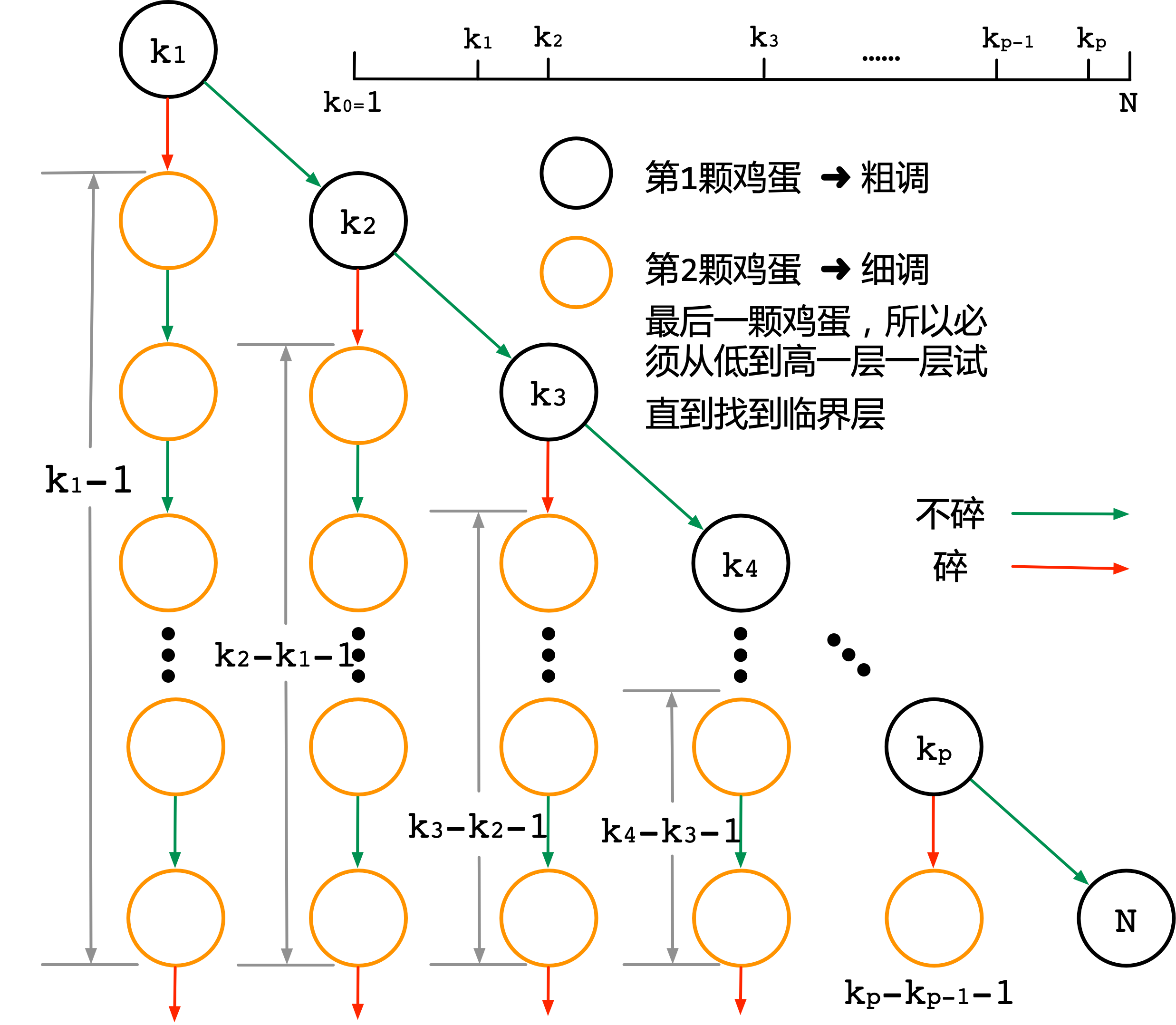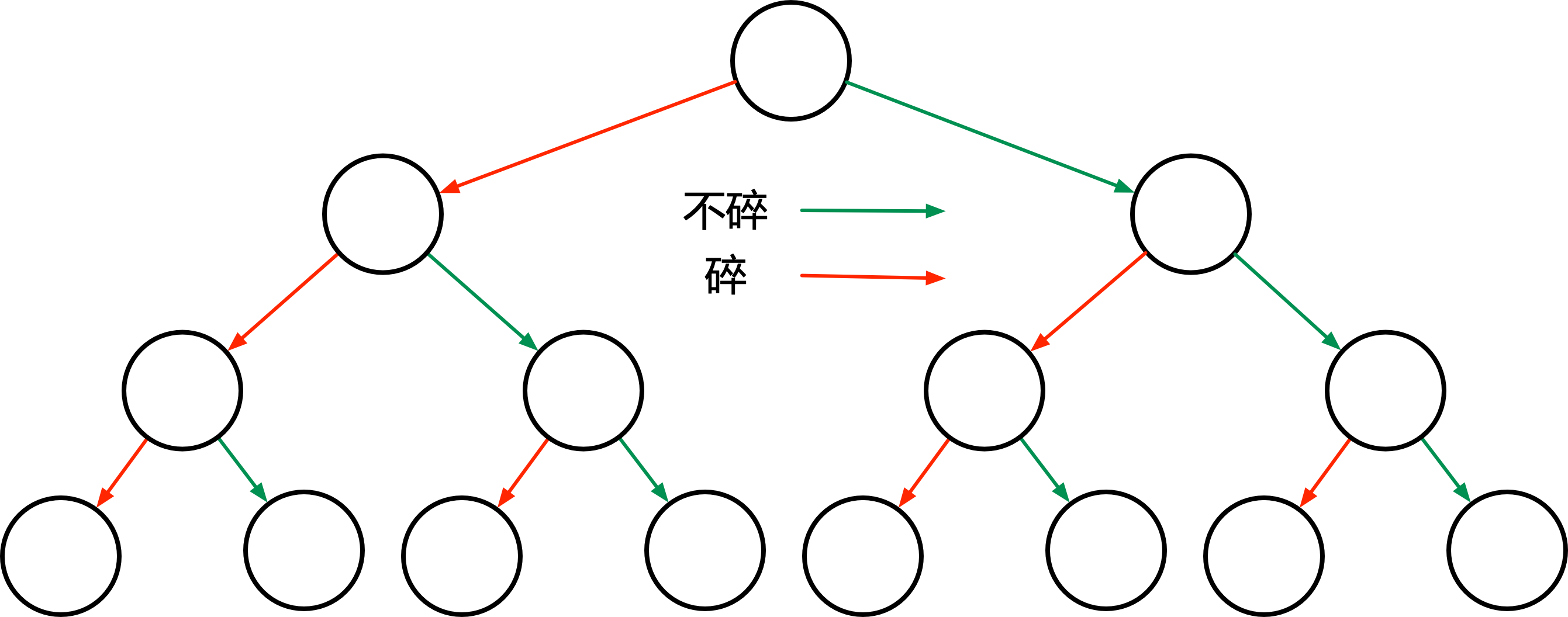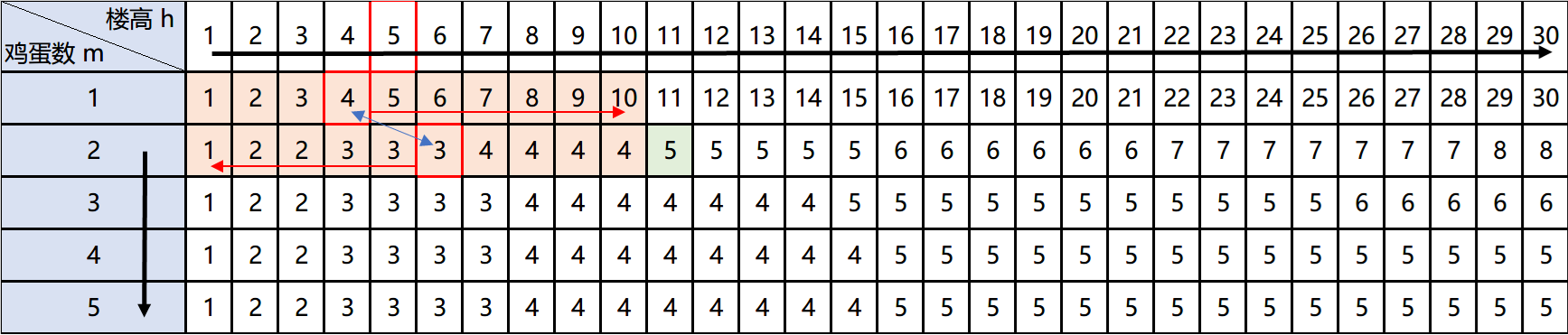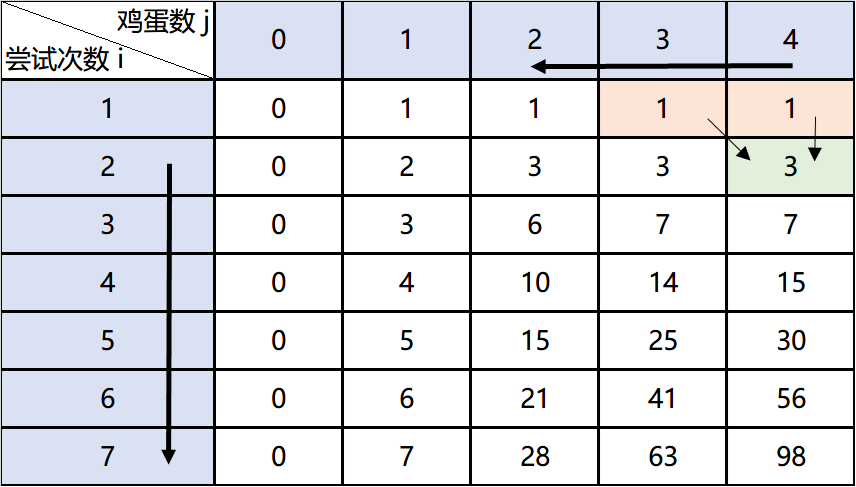【阅读时间】10000+字 | 17 - 22 min

# 1. 题目描述

## 1.1 求什么？

⭐️〔题目要求〕找到 $x$ 层落下不会碎，$x+1$ 层落下会碎的临界层所需要的最少尝试次数 $r$ (r for result)

# 2. 解题思路

## 2.1 一个鸡蛋

$$r = \max_{1 \leqslant x \leqslant N}(\forall S_i(x)) \tag{1}$$

## 2.2 两个鸡蛋

### 2.2.1 粗调和细调

• 没有分叉，一路推理 ➜ 〔线性结构〕
• 看到决策结果有分叉〔树形结构〕
• 若在推理过程中，产生交汇〔图结构〕

### 2.2.2 树形结构〔解释这棵树〕在每一层扔下一个鸡蛋时，都有两种可能，碎了没碎，分别对应了两个子树。一旦碎了，那么就使用1个鸡蛋的问题思路来解题，直到找到临界层。在 $k_1$层没碎，那么需在 $k_2$ 层继续尝试，直到最高层 $N$ 找到临界层（上图的标注不是树高，是灰色图例标识出部分树高

〔输入自变量〕 $k_1, k_2,\cdots,k_p$ 〔待求〕最小的尝试次数（即所有策略中最大值的最小值）等价于➜ 求一个策略组合 $k_1, k_2,\cdots,k_p$，使得树的高度最小

🌰 比如 $k_1 = 50$，意思是在50层扔下第一个鸡蛋

• 如果碎了，那么接下来的尝试方法就是从第1层开始一个一个往上尝试，直到49层，所以我们的答案 r = 50 （不记得如何计算这个值可以参考一个鸡蛋
• 如果没碎，那么第一个鸡蛋继续在 k_2 = 75 层扔，假设还是没碎，继续从 k_3 = 90 层的地方扔下，此时碎了，那么第二课鸡蛋就需要从76层开始，一层一层往上尝试直到89层，需要 89-76+1=24 次，第一个鸡蛋已经扔了3次，最后得到没碎情况下的例子的答案 r=27 次

### 2.2.3 最优解的情况证明

⭐️ 接下里需要确定树的形状（同时也是确定策略的过程）。从直觉来看，如果树的形状倾向于满二叉树，那么树的高度最小。❓ 如何证明这个直觉的正确性呢？既然要计算树高，那么就把每一个叶子节点的树高一个一个列出来
$$\left. \begin{array}{l} k_1 \\ k_2 -k_1 +1 \\ k_3-k_2 +2 \\ \cdots \\ k_p - k_{p-1} +{p-1} \\ N- k_p + p \\ \end{array} \right\} \sum = N + \frac{(1+p)p}{2} \tag{2}$$$$\begin{array} \\ k_1 &= k_2 - k_1 + 1 &\implies &k_2 = 2k_1 - 1 = k_1 + (k_1 - 1) \\ k_2 -k_1 +1 &= k_3-k_2 +2 &\implies &k_3 = 3k_1 -3 = k_1 + (k_1 -1) + (k_1 -2) \\ \cdots \\ k_{p-1} -k_{p-2} +p-2 &= k_{p}-k_{p-1}+ p - 1 &\implies &k_p = \underbrace{ k_1 + (k_1-1) + (k_1-2) + \cdots }_{p项} \end{array} \tag{3}$$

$$k_1 + (k_1-1) + (k_1-2) + \cdots + 1 \sim N \implies \frac{k_1(k_1 + 1)}{2} = N \tag{4}$$

⭐️ 到此为止，2个鸡蛋问题解决：找到了一个明确的函数，利用抽象思维，写出它的表达式 $r = f(2, N)$，而这个 $f()$ 即求方程 $\frac{x(x+1)}{2} = N$ 解 $x$ 的上整数 $\lceil x \rceil$

# 3. 问题拓展

2个鸡蛋100层楼已经解决，深究下去，H层楼M个鸡蛋的问题呢？Leetcode有这道题：887. Super Egg Drop

1个和2个鸡蛋的问题已经解决

② 理解了最大值的最小值在本题中的含义

③ 答案的值只和楼层高度有关，和最低楼层与最高楼层无关

## 3.1 符号化描述

### 〔情况 1〕 碎了

• 1）此时剩下的鸡蛋数，这个非常明显，符号化记为 $m$ 个鸡蛋
• 2）根据2个鸡蛋难题时的结论可知，第二个自变量是此时未知情况楼层的层高（未知情况只楼层范围内还从来没有用鸡蛋尝试过，无法缩小范围），符号化记为 $h$ 层（h for height）

⭐️ 用一个表达式 $r = f(h, m)$ 表示楼高为 $h$ 时，还剩下 $m$ 个鸡蛋时的最少尝试步数

## 3.2 构造逻辑链

1️⃣ 楼高为 $h$ ，剩下 $m$ 个鸡蛋时的最少尝试步数：$f(h, m)$

2️⃣ 在第 $k$ 层扔下1个鸡蛋碎了后的最少尝试步数：$f(k-1, m-1)$

3️⃣ 在第 $k$ 层扔下1个鸡蛋没碎后的最少尝试步数：$f(h-k, m)$

〔推倒 $a$〕考虑到2️⃣3️⃣是一种策略选择后的可能性，根据上面的分析（最大值的最小值原理）两种策略中临界层 $x$ 可能在任何一层。遍历所有 $x$ 的情况时，最终尝试步数的结果是其中的最大值，所以选择2️⃣3️⃣中值更大的那个

〔推倒 $b$〕1️⃣需要仍一次鸡蛋，尝试步数 $+1$

〔推倒 $c$〕$k$ 是一个自变量，在这个情况下，可取到 $[1, h)$ 中的任意值，⭐️假设2️⃣3️⃣已知，只要在定义域内穷举出所有 $k$ 的可能值，并找出最大尝试步数中的最小值即可

$$f(h, m) = \underbrace{\min_{1 \leqslant k \leqslant h} {\overbrace{\overbrace{\max [f(k-1, m-1), f(h-k,m)]}^{a}+1}^{b}}}_{c}\tag{5}$$

### 3.2.2 边界条件

$f(h, 1) = h$ ，当剩余鸡蛋数为1的时候，答案为现在的楼高

$f(0, m) = 0$ ，当楼高为0时，无法尝试，答案记为0 ，或者 $f(1,m) = 1$，表示楼高为1时，最小尝试次数为1

## 3.3 动态规划

• 〔拆分思维〕拆分问题的能力。大问题化小问题，无后效性，当前状态与未来状态无关。
• 〔抽象思维〕使用状态来描述问题的能力，或说用抽象符号或状态机来描述问题的能力
• 〔归纳思维〕数学归纳法，逻辑推导能力。由 $\lt i$ 的所有状态推倒出 $i$ 时刻状态的能力

# 4. 实现优化和分析

## 4.1 基础版

🔄〔优化〕Recursive（递归）的写法虽然清晰明了，但在工程上有调用函数的开销，系统栈深度限制等弊端，所以最好写成循环版本，否则在工作中可能返工

## 4.2 循环版本

🔄〔优化〕分析一下时间复杂度和空间复杂度，时间复杂度为 $O(mh^2)$ ，空间复杂度为 $O(hn)$ ，很明显，空间上可以优化到 $O(h)$，原因是状态转移方程只和 $m$ 与 $m-1$ 有关，使用两个数组滚动即可

## 4.3 空间优化版本

🔄〔优化〕这里需要使用数据结构中折半查找判定树理论。假设我们对鸡蛋的数量不做限制，那么这棵树🌰 如果还是不明白，上终极杀招，举例子。假设16层楼，但有4个鸡蛋。根本不用设计，二分查找即可，效率肯定最高。比如16层楼，只要鸡蛋数大于4，最大尝试次数就是4，可以直接算出答案

## 4.4 下界优化🔄 观察发现，有一个很重要的规律，即单调性，写成公式为 $f(m, h) \geqslant f(m, h-1)$ 当 $h \geqslant 1$ 。如果满足单调性，那么又可以在搜索时使用二分查找。先姑且不考虑如何证明单调性，工程上，在资源允许的情况下，先尝试，看看能不能得到正确结果（用正确算法和这个尝试算法进行验证）直到验证在很大的定义域内都正确，可大胆猜测这个猜测性质是对的（不严谨，但效率较高）

## 4.5 单调性优化

🔄 结果的简单特性部分挖掘完了，继续观察上面的状态转移图，发现很多项是相等的，那么可不可以找到，在某种条件满足时，状态可以直接推出，而不用进行 $k$ 的遍历搜索？这个时候就需要从状态转移方程本身入手是挖掘其中的数学特性，这部分不是非常关键，较难，可直接跳过

$$\begin{array}& f(h, m) = \min\limits_{1 \leqslant k \leqslant h} \{\max [f(k-1, m-1), f(h-k,m)]+1\} \\ f(h, m) \leqslant \max [f(k-1, m-1), f(h-k,m)]+1 \quad(1 \leqslant k \leqslant h) \\ 令\; k = 1 \implies f(h, m) \leqslant f(h - 1, m) + 1 \end{array} \tag{6}$$

• 若某个决策 $k$ 可使得 $f(h - 1, m) = f(h, m)$ ，则一定 $f(h, m) = f(h-1, m)$

• 若所有决策 $k$ 都不能使 $f(h - 1, m) = f(h, m)$ ，则一定 $f(h, m) = f(h - 1, m) + 1$

## 4.7 不同角度看问题

### 4.7.3 状态转移方程

• 〔碎了〕那么在后面的 $i-1$ 次里，需用 $j-1$ 个鸡蛋在下面的楼层中确定楼高。为了使 $h(i,j)$ 达到最大，希望下面的楼层数（楼高）最大，根据状态定义，记为 $h(i-1, j-1)$
• 〔没碎〕那么在后面的 $i-1$ 次里，需用 $j$ 个鸡蛋在上面的楼层中确定楼高。同理，需要楼层数达到最大，记为 $h(i-1,j)$

### 4.7.5 继续优化# 5. 工程思维总结和感悟

## 5.1 工程思维

⭐️ 这一部分是【直观算法】系列的最终目的。求同遵异，寻找每一道算法题背后的工程思维，提升自己真正解决工程问题的能力。博主对自己的定位是【机器学习+区块链产品经理】，但同时对技术也有好奇心，喜欢钻研，才开坑写【直观算法】系列

### 5.1.2 等价思维

⭐️ 学习的真正本质是在一个领域内不断的去建立这种沟通共识的框架。举个例子，学过线性代数的人，只要提到矩阵，就能想到变换。这就是都学过线性代数的人交流密码，如矩阵的逆 = 一个具体的反向变换。没学过这门课的人是无法和学过的人进行沟通的。这也从很大程度加强了人们沟通的效率，所以，学习带来的终极成效之一其实是降低了人类社会的沟通时间，提高了效率

## 5.2 工程应用

🌰 如果要找学科分类，鸡蛋难题属于运筹学问题，典型应用是破坏性试验，如测试汽车、飞机、火箭等的若干极限性能。举个例子，汽车保全乘客安全的最高碰撞末速，那么为了保证能找这个值，一定会用类似的思路来解决问题。

【参考文献】